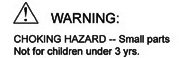# Math Manipulatives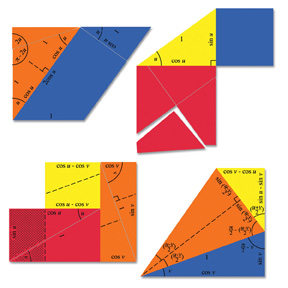Hands-on Trigonometry Proofs \$29.95 This set contains four separate "proofs" of trigonometry relations, with examples of a Pythagorean identity, a sum formula, a double-angle formula, and two sum-to-product formulas. Demonstration of each relation is accomplished by physically rearranging the pieces to establish equalities of areas or lengths. These are not rigorous mathematical proofs, but rather visual common-sense proofs. As such, they help make these abstract relationships real and believable. By actually handling the pieces, students engage their minds to a greater degree than they would by merely looking at the proofs on paper. The color instructions describe how each proof is demonstrated, and four reproducible worksheets reinforce the material. Contains 20 magnetic foam pieces. Grades 7-12.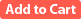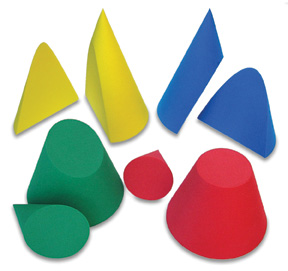Conic Sections \$29.95 This set contains four cones cut four different ways to reveal the different conic sections - circle, ellipse, parabola, and hyperbola. Four reproducible worksheets cover various properties of the conic sections, including their descriptions using Cartesian and polar coordinates, their foci, and their eccentricity. Cones measure 2-3/4" across the base and 4" in height. Grades 7-12.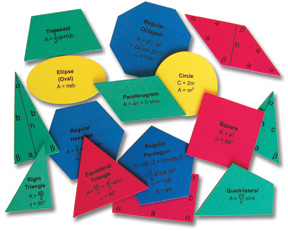Advanced Geometric Shapes \$17.95 Fifteen different foam geometric shapes. Angles and edges are labeled on the frontsides to facilitate classroom discussion, both with and without the use of trigonometric functions. The names of the shapes and their areas are printed on the backsides, allowing the shapes to be used as flashcards. The set includes four worksheets for classroom use. The thickness of the pieces is 0.2" (0.5 cm), and the circle diameter is 4" (10 cm).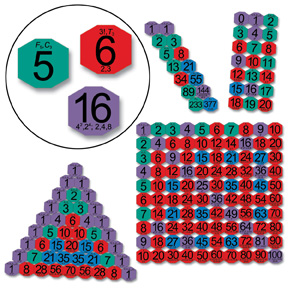Pattern Numbers \$29.95 This set teaches about the properties of numbers and uses colored tiles to illustrate patterns formed by numbers. Colors indicate which numbers are squares, primes, even numbers that aren't squares, and odd numbers that are composite (not prime). The individual tiles contain information about the number on the tile, including its factors and whether it is a factorial, triangular, Fibonacci, or Catalan number. The tiles are shaped to allow vertical alignment of rows or staggered rows for optimal display of various configurations: counting by different numbers, the multiplication table, Pascal's triangle, triangular numbers, Fibonacci numbers, and more. Contains 186 magnetic foam tiles, color instructions, and eight reproducible worksheets. Grades K-12.